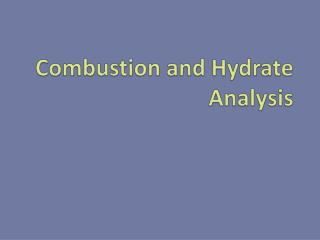DownloadDownload PresentationCombustion and Hydrate Analysis

# Combustion and Hydrate Analysis

Télécharger la présentation## Combustion and Hydrate Analysis

- - - - - - - - - - - - - - - - - - - - - - - - - - - E N D - - - - - - - - - - - - - - - - - - - - - - - - - - -
##### Presentation Transcript

1. Combustion and Hydrate Analysis

2. Agenda • Review • Combustion Analysis • Practice Problem • Hydrate Analysis • Practice Problem

3. Review • What is the law of multiple proportions? • What two kinds of formulas did we learn about? • Describe one way to determine the empirical formula.

4. Combustion Analysis • Hydrocarbons are an important and diverse group of compounds • Chemists can determine the % composition by combustion analysis • Used anywhere unknown compounds need to be analyzed!

5. How It Works! • Hydrocarbons are burned in pure oxygen • Carbon dioxide and water are produced • CuO oxidizes unreacted C and CO to make CO2 • All H becomes H2O and all C becomes CO2 • H2O and CO2 are absorbed separately • Masses of each are used to determine % composition

6. Sample Problem • A 1.00g sample of a pure compound, containing only carbon and hydrogen, was combusted in a carbon-hydrogen combustion analyzer. The combustion produced 0.619g of water and 3.338g of carbon dioxide. a) Calculate the masses of carbon and hydrogen in the sample. b) Find the empirical formula of the compound.

7. Hydrate Analysis • Hydrates are ionic compounds with water incorporated into their crystal structure • Anhydrous compounds have no water attached • Compare MgSO47H2O and MgSO4 • The molar mass and the % composition must include the bound water molecules H2O

8. Hydrate Analysis • How could hydrates be converted into anhydrous compounds? • How could you use this to determine the number of molecules of water bound to the salt? HEAT!

9. Sample Problem • A hydrate of barium hydroxide, Ba(OH)2·xH2O, is used to make barium salts and to prepare certain organic compounds. Since it reacts with CO2 from the air to yield barium carbonate, BaCO3, it must be stored in tightly stoppered bottles. • A 50g sample of the hydrate contains 27.2g of Ba(OH)2. Calculate the percent, by mass, of water in Ba(OH)2·xH2O. • Find the value of x in Ba(OH)2·xH2O.